«

# emlog 开发一个小插件（截取正文生成 xx 字摘要）的过程

## emlog 系统的插件是怎么运行的？

### 样板程序 tips 解析

#### 精简代码

/content/plugins/tips/tips.php

<?php
/*
Plugin Name: 小贴士
Version: 2.0
Plugin URL:
Description: 这是世界上第一个emlog插件，它会在你的管理页面送上一句温馨的小提示。
Author: emlog官方
Author URL: https://www.emlog.net
*/

!defined('EMLOG_ROOT') && exit('access deined!');

$array_tips = [ '为防文章丢失，emlog会在你书写文章的时候为你自动保存', '你可以把你未写完的文章保存到草稿箱里', '大尺寸的图片上传时会自动生成缩略图，从而加快页面加载速度', '请关注后台首页的官方信息栏目，这里有最新的动态', '你可以把图片嵌入到内容中，让你的文章图文并茂', '你可以在写文章的时候为文章设置访问密码，只让你授予密码的人访问', 'emlog支持多人联合撰写', 'emlog支持自建页面，并且可以上传照片，为自己做一个图文并茂的自我介绍页吧', '新建一个允许发表评论的页面，你会发现其实它还是一个简单的留言板', '检查你的站点目录下是否存在安装文件：install.php，有的话请删除它', '使用chrome浏览器，更好的体验emlog', '从明天起，做一个幸福的人', ]; function tips() { global$array_tips;
$i = mt_rand(0, count($array_tips) - 1);
$tip =$array_tips[$i]; echo "<div id=\"tip\">$tip</div>";
}

function tips_css() {
echo "<style type='text/css'>
#tip{
background:url(../content/plugins/tips/icon_tips.gif) no-repeat left 3px;
margin:5px 0px;
font-size:12px;
color:#999999;
}
</style>\n";
}

addAction('adm_head', 'tips_css');

<?php
/*
Plugin Name: 小贴士
Version: 2.0
Plugin URL:
Description: 这是世界上第一个emlog插件，它会在你的管理页面送上一句温馨的小提示。
Author: emlog官方
Author URL: https://www.emlog.net
*/

!defined('EMLOG_ROOT') && exit('access deined!');

function tips() {
echo "<div id=\"tip\"> 你好，我是一个插件！</div>";
}



#### 顶部注释信息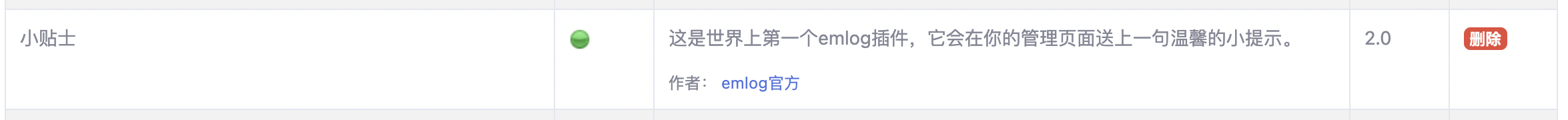#### 鉴定访问权限

!defined('EMLOG_ROOT') && exit('access deined!');

-------------------------------------------------------------------------------------
tips.php
-------------------------------------------------------------------------------------

<?php
/*
Plugin Name: 小贴士
Version: 2.0
Plugin URL:
Description: 这是世界上第一个emlog插件，它会在你的管理页面送上一句温馨的小提示。
Author: emlog官方
Author URL: https://www.emlog.net
*/

!defined('EMLOG_ROOT') && exit('access deined!');

const TIPS_AAR = '';
require 'tips_word.php';

function tips() {
echo tips_getWord();
}

-------------------------------------------------------------------------------------
tips_word.php
-------------------------------------------------------------------------------------

<?php

!defined('TIPS_AAR') && exit('access deined!');

function tips_getWord(){
return "从明天起，做一个幸福的人";
}


#### 动作函数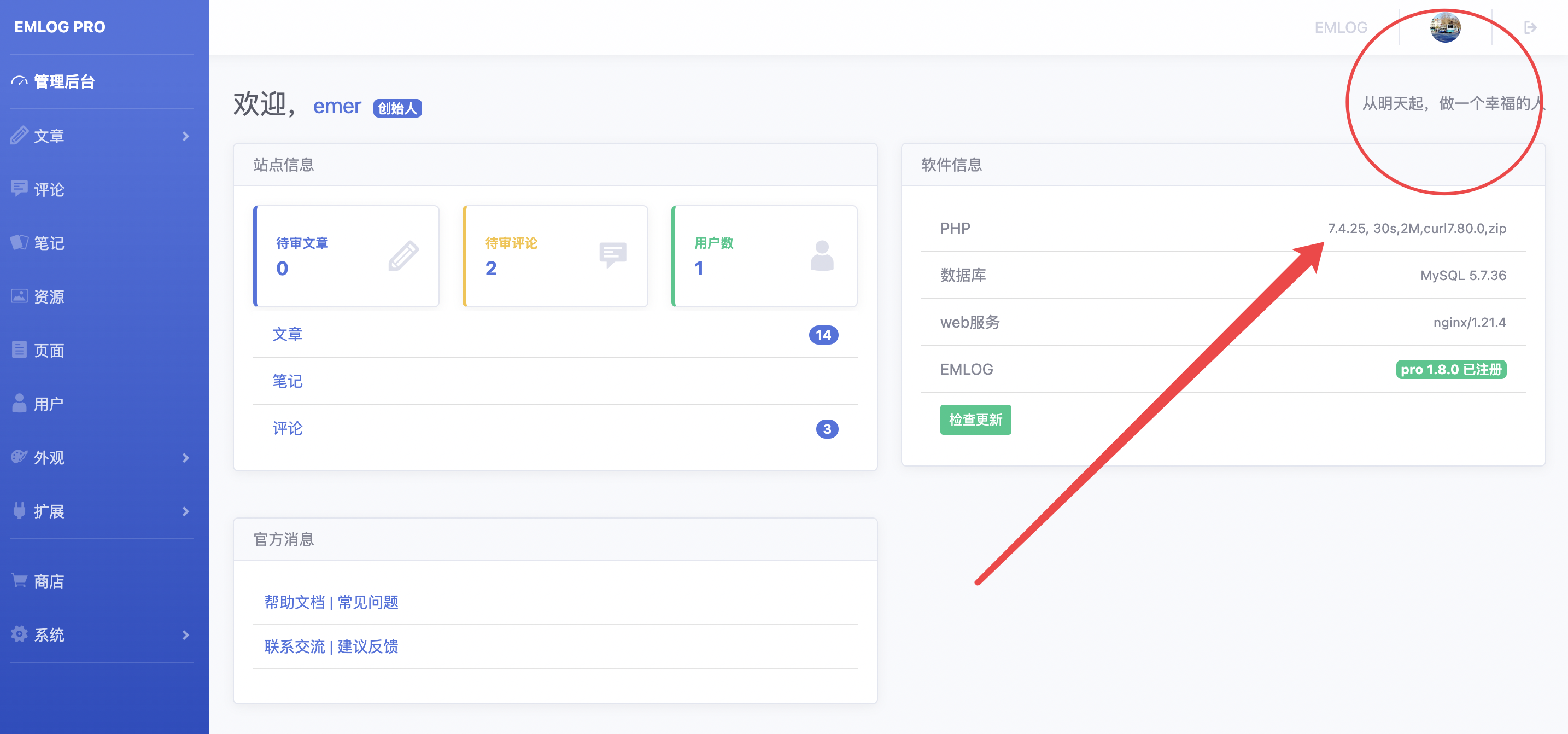addAction('adm_main_top', 'tips');

https://www.emlog.net/docs/#/plugin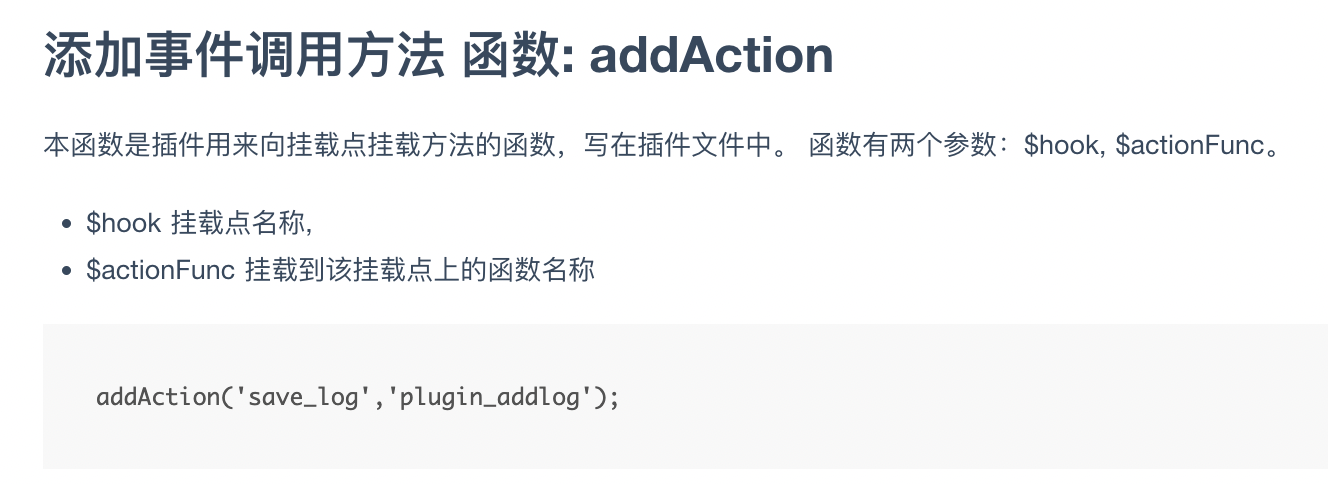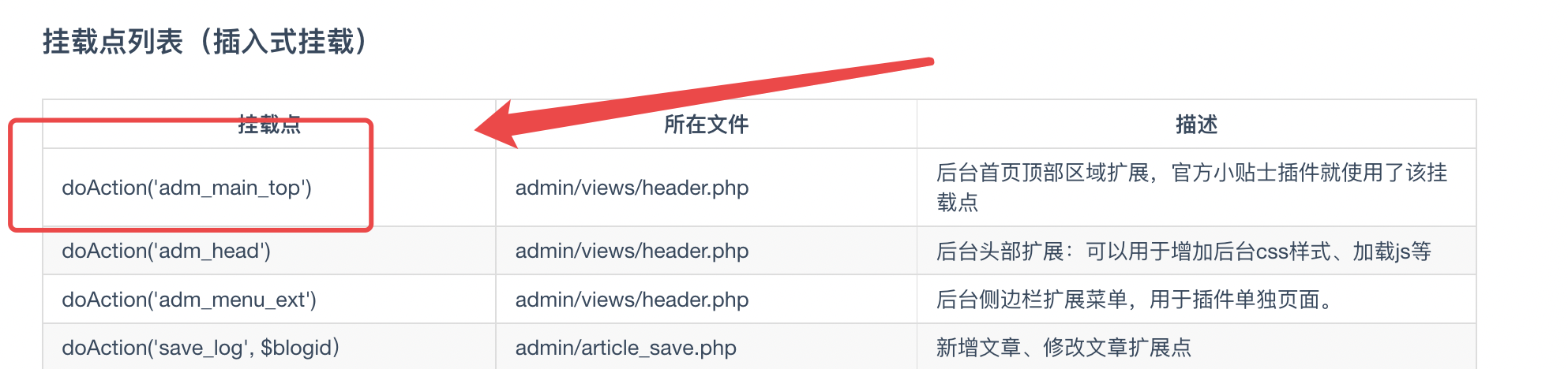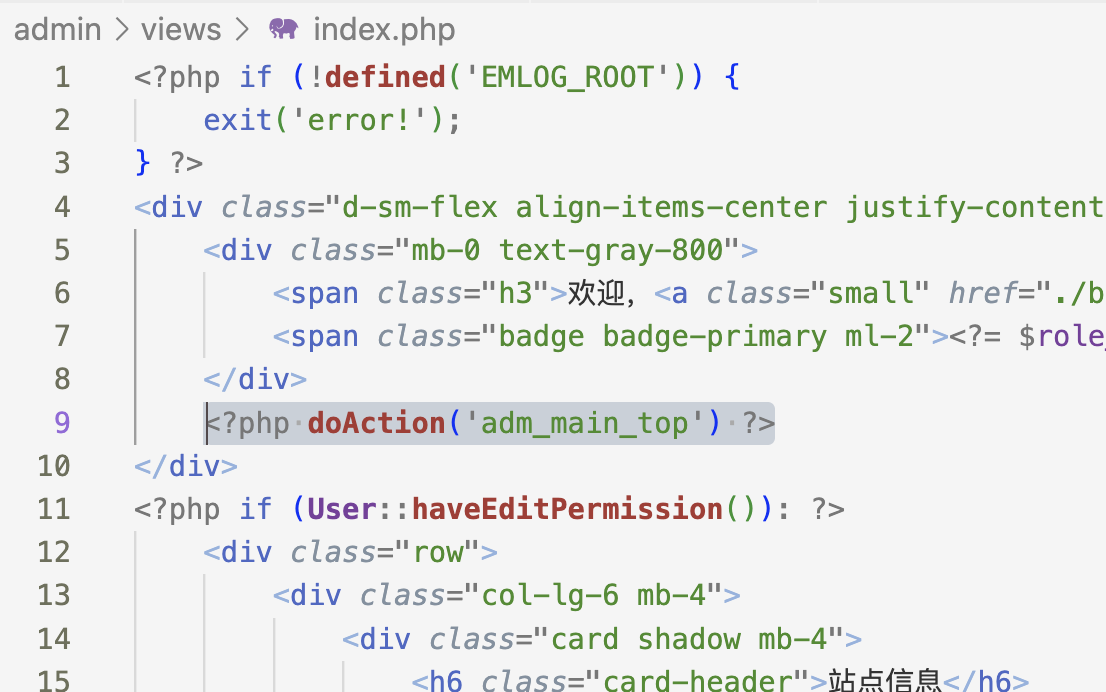## 开始制作我们的插件！

### 初始化开发环境

/clicksum
|----clicksum.php     # 插件的主文件
|----clicksum_setting.php     # 插件的后台设置
|----clicksum_callback.php  # 插件初始化（可用于写入默认配置）

-------------------------------------------------------------------------------------
clicksum.php
-------------------------------------------------------------------------------------
<?php
/*
Plugin Name: 一键生成摘要
Version: 1.0
Plugin URL:
Description: 在文章编辑页，一键生成摘要。可自定义设置摘要字数（如 200 字）。
Author:
Author URL:
*/

!defined('EMLOG_ROOT') && exit('access deined!');

function clicksum_display() {
echo "";
}

-------------------------------------------------------------------------------------
clicksum_setting.php
-------------------------------------------------------------------------------------
<?php
!defined('EMLOG_ROOT') && exit('error');

function plugin_setting_view(){
?>

<div>hi</div>

<?php } ?>

-------------------------------------------------------------------------------------
clicksum_callback.php
-------------------------------------------------------------------------------------
<?php
!defined('EMLOG_ROOT') && exit('error');


function clicksum_display() {
}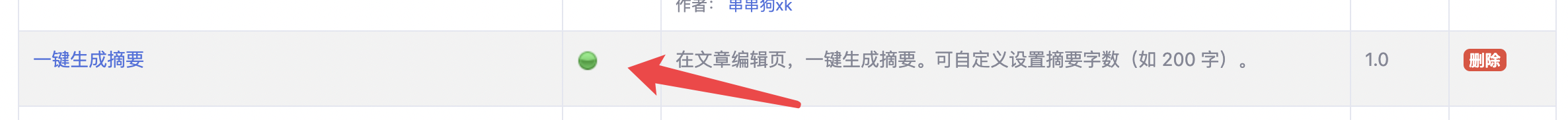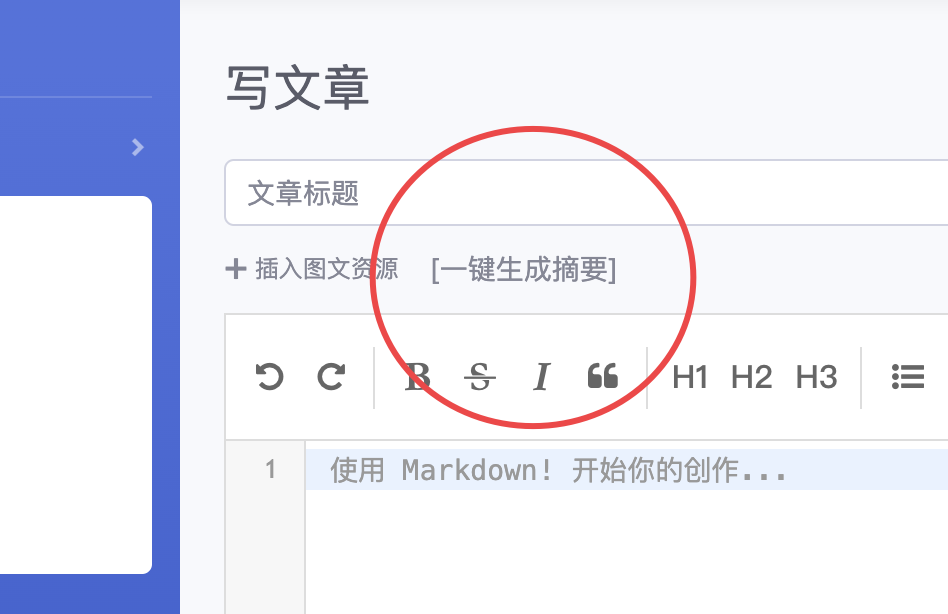!defined('EMLOG_ROOT') && exit('access deined!');

function clicksum_display() {

echo <<<EOF

<script>
</script>

EOF;
}

addAction('adm_writelog_head', 'c_display');

### 研究如何在编辑器插入内容

http://editor.md.ipandao.com/examples/set-get-replace-selection.html

var testEditormd;  // 定义编辑器
$(function() { testEditormd = editormd("test-editormd", { // 生成编辑器 width: "90%", height: 640, path : '../lib/' }); }); testEditormd.setCursor({line:1, ch:2}); // 设置光标位置为 1 行 2 列 testEditormd.insertValue("????"); // 在光标位置插入内容“????” 因此，我们可写这样的代码，向摘要编辑器里输入 hello world。  echo <<<EOF <script> Editor_summary.setCursor({line:0, ch:0}); // 让光标放在最开始处 Editor_summary.insertValue("hello"); // 在光标位置插入内容“????” </script> EOF; 好，显示效果如下。现在我们就可以在插件里对各个编辑器为所欲为了！！！首先我们要获取文章编辑器的内容。根据 editor.md 的官方文档，我们可以使用 .getPreviewedHTML() 函数来获取。当然，直接获取 class="editormd-preview-container" 的 html 也可以。 之后就是思考程序流水线了。我们获取的是 editor.md 的 html 源代码。那么我们可以设计下面这样的流水线来生成摘要了。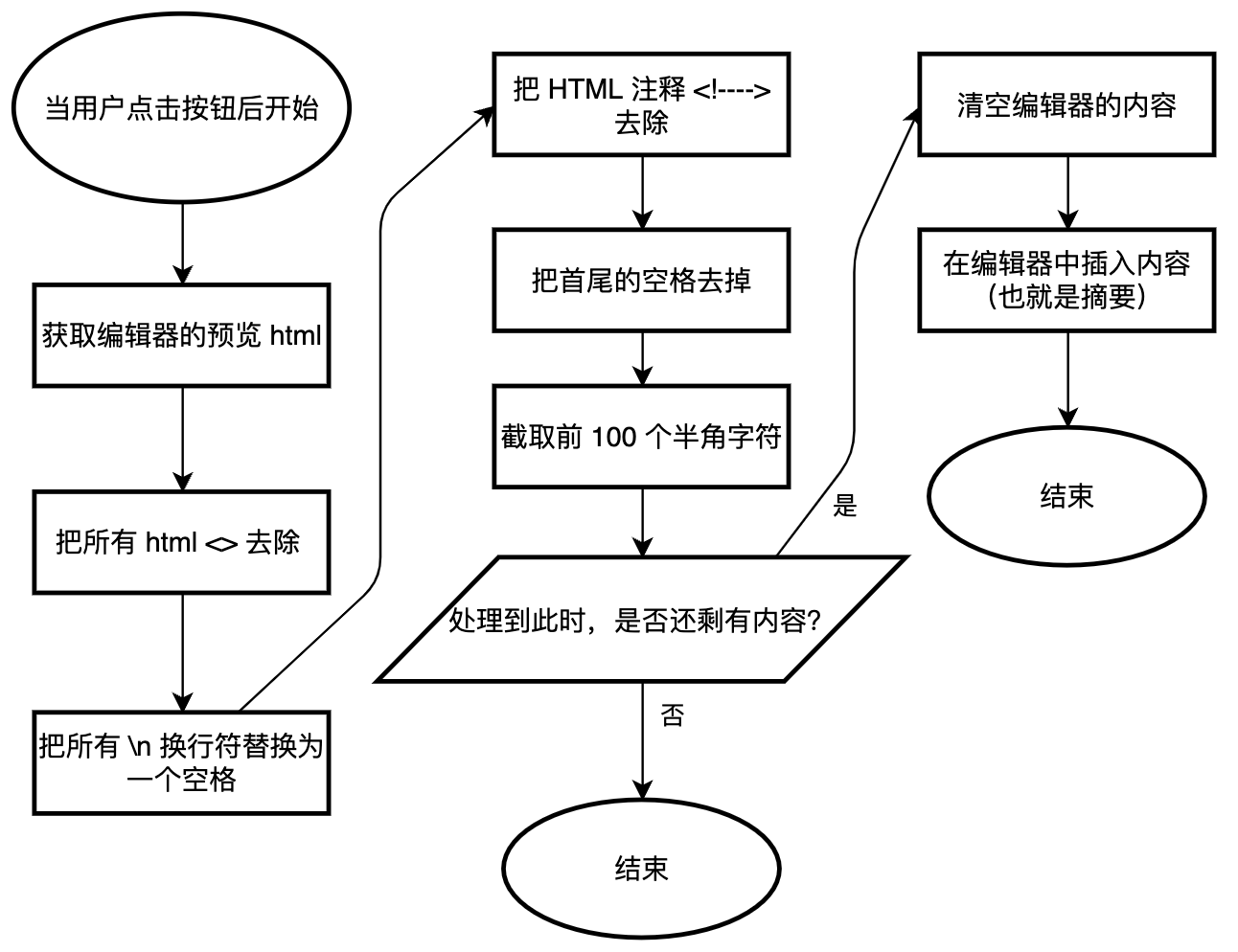JavaScript 比较简单，就不多说了，如下述代码。 function clickSum(){ const pattern1 = /<(\S*?)[^>]*>.*?/ig; const pattern2 = /\\n/ig; const pattern3 = /\\\x3C!--.*-->/ig; const pattern4 = /^\s+/ig; const pattern5 = /\s+$/ig;

let endText = "$clicksum_string"; // 摘要的结尾要加上的字符串 const number =$clicksum_number;  // 截取的字符数

let articleHtml = Editor.getPreviewedHTML()
let articleText = articleHtml.replace(pattern1, "");  // 去除 html 的尖括号
articleText = articleText.replace(pattern2, " ");  // 换行符变空格
articleText = articleText.replace(pattern3, "");  // 去除 HTML
articleText = articleText.replace(pattern4, "");  // 这两行去除首尾空格
articleText = articleText.replace(pattern5, "");

if(articleText.length > articleText.substring(0,number).length){
articleText = articleText.substring(0,number);
} else {
endText = '';
}

if (articleText.length === 0 ) return  // 如果处理到此时，内容为空，退出

/* 清空编辑器里之前的内容 */
Editor_summary.setCursor({line:0, ch:0});  // 光标放在开头
Editor_summary.setSelection({line:0, ch:0}, {line:(999*999*999), ch:(999*999*999)});  // 内容全选
Editor_summary.replaceSelection("");  // 清空

Editor_summary.insertValue(articleText + endText);

return
}

clickSum();
})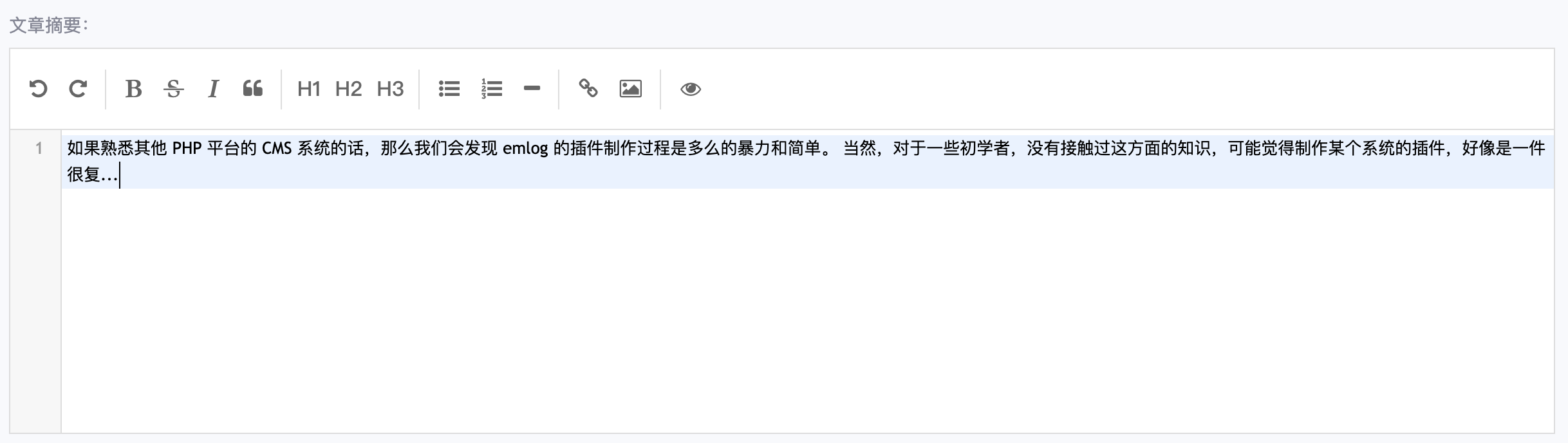1. 后台可设置截取的字符数，比如 200、300
2. 后台可设置是否在提交时自动截取
3. 后台可设置摘要后的添加的字符串内容（即更改 ... 为其他内容）
4. 后台可设置是否在生成摘要后，让编辑器预览。

### 插件后台设置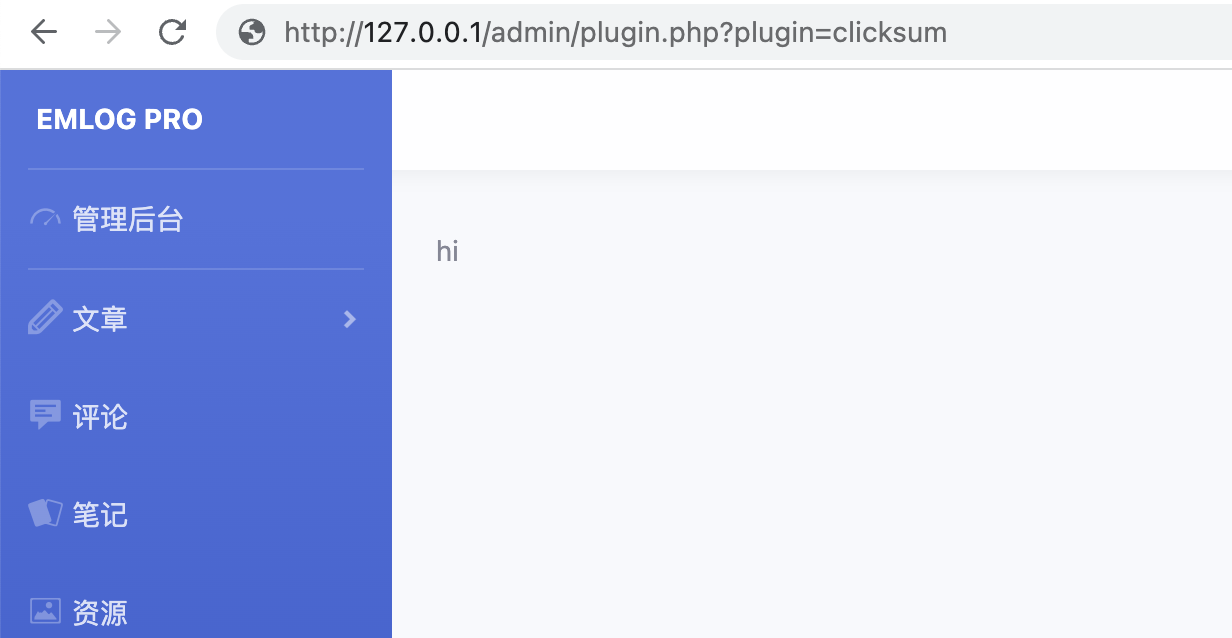<?php
!defined('EMLOG_ROOT') && exit('error');

function plugin_setting_view(){
?>

<form action="plugin.php?plugin=clicksum&act=save" method="post" name="input">
<div>
<label for="input1">提交文章时自动提交摘要：</label>
</div>
<div>
<label for="input2">生成摘要后，编辑器预览：</label>
</div>
<div>
<label for="input3">截取的字符数：</label>
<input type="number" id="input3" name="number" required />
</div>
<div>
<label for="input4">摘要后接字符串：</label>
<input type="text" id="input4" name="string" required />
</div>
<input type="submit" value="保存" />
<form>

<?php } ?>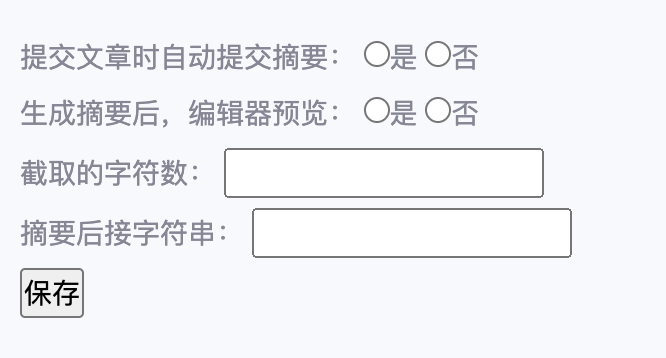### 获取数据


function plugin_setting_view(){

$act = isset($_GET['act']) ? addslashes($_GET['act']) : ''; if($act == "save") {
$getConfig = [ // 获取 post 得来的数据 'autogen' => isset($_POST['autogen']) ? addslashes($_POST['autogen']) : 'n', 'autopre' => isset($_POST['autopre']) ? addslashes($_POST['autopre']) : 'n', 'number' => isset($_POST['number']) ? addslashes($_POST['number']) : '100', 'string' => isset($_POST['string']) ? addslashes($_POST['string']) : '...' ]; }  当我们的 get 请求里有 act=save 时，就来获取数据。如上图所示，把数据储存到$getConfig 这个对象里。

### 超级方便的 emlog Storage

Pro 版本以后，我们可以使用 emlog Storage（emlog 插件的数据存储器）来存储我们的插件数据了，直接和 emlog 的数据库表融为一体，方便很多。

储存数据

$plugin_storage = Storage::getInstance('plugin_name');//使用插件的英文名称初始化一个存储实例$plugin_storage->setValue('key', 'xxxxxx'); // 设置key的值为 xxxxxx

$plugin_storage = Storage::getInstance('plugin_name'); //使用插件的英文名称初始化一个存储实例$ak = $plugin_storage->getValue('key'); // 读取key值 因此，我们可在我们的代码中使用这个功能来将我们的配置储存到 emlog 的数据表里。 应注意，任何用户输入的数据都必须必须必须必须必须必须必须使用 addslashes()_ 等措施来进行 HTML 过滤，否则将会带来极大的安全隐患！ $clicksum_storage = Storage::getInstance('plugin_clicksum');  // 初始化 emlog 插件存储

function plugin_setting_view(){
global $clicksum_storage;$act = isset($_GET['act']) ? addslashes($_GET['act']) : '';

if($act == "save") {$getConfig = [  // 获取 post 得来的数据
'autogen'   => isset($_POST['autogen']) ? addslashes($_POST['autogen']) : 'n',
'autopre'   => isset($_POST['autopre']) ? addslashes($_POST['autopre']) : 'n',
'number'    => isset($_POST['number']) ? addslashes($_POST['number']) : '100',
'string'    => isset($_POST['string']) ? addslashes($_POST['string']) : '...'
];

$clicksum_storage->setValue('autogen',$getConfig['autogen']);
$clicksum_storage->setValue('autopre',$getConfig['autopre']);
$clicksum_storage->setValue('number',$getConfig['number']);
$clicksum_storage->setValue('string',$getConfig['string']);
}

### 后台显示与配置数据库同步

function plugin_setting_view(){
global $clicksum_storage;$act = isset($_GET['act']) ? addslashes($_GET['act']) : '';

if($act == "save") {$getConfig = [  // 获取 post 得来的数据
'autogen'   => isset($_POST['autogen']) ? addslashes($_POST['autogen']) : 'n',
'autopre'   => isset($_POST['autopre']) ? addslashes($_POST['autopre']) : 'n',
'number'    => isset($_POST['number']) ? addslashes($_POST['number']) : '100',
'string'    => isset($_POST['string']) ? addslashes($_POST['string']) : '...'
];

$clicksum_storage->setValue('autogen',$getConfig['autogen']);
$clicksum_storage->setValue('autopre',$getConfig['autopre']);
$clicksum_storage->setValue('number',$getConfig['number']);
$clicksum_storage->setValue('string',$getConfig['string']);
}

function autogen_check($key){ global$clicksum_storage;

if ($clicksum_storage->getValue('autogen') ==$key){
return "checked";
}
}

function autopre_check($key){ global$clicksum_storage;

if ($clicksum_storage->getValue('autopre') ==$key){
return "checked";
}
}

function number_content(){
global $clicksum_storage; return$clicksum_storage->getValue('number');
}

function string_content(){
global $clicksum_storage; return$clicksum_storage->getValue('string');
}

?>

<form action="plugin.php?plugin=clicksum&act=save" method="post" name="input">
<div>
<label for="input1">提交文章时自动提交摘要：</label>
<input type="radio" name="autogen" value="y" <?= autogen_check("y") ?> />是
<input type="radio" name="autogen" value="n" <?= autogen_check("n") ?> />否
</div>
<div>
<label for="input2">生成摘要后，编辑器预览：</label>
<input type="radio" name="autopre" value="y" <?= autopre_check("y") ?> />是
<input type="radio" name="autopre" value="n" <?= autopre_check("n") ?> />否
</div>
<div>
<label for="input3">截取的字符数：</label>
<input type="number" id="input3" name="number" value="<?= number_content() ?>" required />
</div>
<div>
<label for="input4">摘要后接字符串：</label>
<input type="text" id="input4" name="string" value="<?= string_content() ?>"required />
</div>
<input type="submit" value="保存" />
<form>

<?php } ?>

### 文章提交时自动生成摘要

$clicksum_storage = Storage::getInstance('plugin_clicksum'); 然后在 clicksum.php 文件下部加上 function clicksum_autoSum(){ echo <<<EOF <script> document.querySelector("#post_button [type='submit']").addEventListener("click", function(){ clickSum(); }); </script> EOF; } if($clicksum_storage->getValue('autogen') == 'y'){
}


<?php
/*
Plugin Name: 一键生成摘要
Version: 1.0
Plugin URL:
Description: 在文章编辑页，一键生成摘要。可自定义设置摘要字数（如 200 字）。
Author:
Author URL:
*/

!defined('EMLOG_ROOT') && exit('access deined!');

$clicksum_storage = Storage::getInstance('plugin_clicksum'); // 初始化 emlog 插件存储 function clicksum_display() { global$clicksum_storage;

$clicksum_number =$clicksum_storage->getValue('number');
$clicksum_string =$clicksum_storage->getValue('string');
$clicksum_preScript = ($clicksum_storage->getValue('autopre') == 'y') ? 'Editor_summary.watch()' :'';

echo <<<EOF

<script>
function clickSum(){
const pattern1 = /<(\S*?)[^>]*>.*?/ig;
const pattern2 = /\\n/ig;
const pattern3 = /\\\x3C!--.*-->/ig;
const pattern4 = /^\s+/ig;
const pattern5 = /\s+$/ig; let endText = "$clicksum_string";  // 摘要的结尾要加上的字符串
const number = $clicksum_number; // 截取的字符数 let articleHtml = Editor.getPreviewedHTML() let articleText = articleHtml.replace(pattern1, ""); // 去除 html 的尖括号 articleText = articleText.replace(pattern2, " "); // 换行符变空格 articleText = articleText.replace(pattern3, ""); // 去除 HTML articleText = articleText.replace(pattern4, ""); // 这两行去除首尾空格 articleText = articleText.replace(pattern5, ""); if(articleText.length > articleText.substring(0,number).length){ articleText = articleText.substring(0,number); } else { endText = ''; } if (articleText.length === 0 ) return // 如果处理到此时，内容为空，退出 /* 清空编辑器里之前的内容 */ Editor_summary.setCursor({line:0, ch:0}); // 光标放在开头 Editor_summary.setSelection({line:0, ch:0}, {line:(999*999*999), ch:(999*999*999)}); // 内容全选 Editor_summary.replaceSelection(""); // 清空 Editor_summary.insertValue(articleText + endText);$clicksum_preScript

return
}

clickSum();
})
</script>

EOF;
}

// 在发布文章时自动生成摘要

function clicksum_autoSum(){
echo <<<EOF

<script>
clickSum();
});
</script>

EOF;
}

if($clicksum_storage->getValue('autogen') == 'y'){ addAction('adm_footer', 'clicksum_autoSum'); } ### 安装插件时设置默认值 现在有一个问题，插件刚开始安装时，是没有初始内容的。可能会有报错的风险，所以需要在插件安装，但还未启用时，进行初始化，也就是向 emlog Stroage 中写入默认配置。 在 emlog 开发中有如下说明。 插件的激活与关闭 在emlog后台的插件管理中,点击每个插件后的状态按钮即可激活/关闭插件。 自emlog4.0.0起增加激活和关闭插件函数，来完成激活和关闭时的一些初始化工作。 如果插件需要，可以给插件增加 plugin_callback.php 文件，其中包含两个函数： • callback_init()为插件激活时调用 • callback_rm()为插件关闭时调用 因此，我们在 clicksum_callback.php 中写入如下内容。 <?php !defined('EMLOG_ROOT') && exit('error'); function callback_init() {$clicksum_storage = Storage::getInstance('plugin_clicksum');

$clicksum_storage->setValue('autogen', 'n');$clicksum_storage->setValue('autopre', 'n');
$clicksum_storage->setValue('number', '100');$clicksum_storage->setValue('string', '...');
}@五哥：没错，plugin_setting() 是插件获取数据的函数，不过很少有人用它（因为群主没有在开发文档里写，甚至 plugin_setting_view() 也没写）。

function plugin_setting_view(){

$action = isset($_GET['action']) ? addslashes($_GET['action']) : ''; if ($action == 'setting') {
if (!empty(\$_POST)) {
// ...获取数据和把数据写入到数据库或其他地方的操作
}
}
}

/* 差不多等价于 */

function plugin_setting(){
// ...获取数据和把数据写入到数据库或其他地方的操作
}@独元殇：好的，谢谢，最近在学写插件，有问题再请教@五哥：好滴<(￣︶￣)>ange 2023-01-29 16:13Lvtu 2022-11-10 11:59@Lvtu：......我一直以为你博客上的摘要都是模版或系统自己生成的，原来也是自己手复制粘贴的。Lvtu 2022-11-12 15:46
@独元殇：嗯，都是写好正文后复制粘贴的！@沉舟侧畔：PHP 现在当玩具挺好，上手很快，网上资料非常非常多。不过很可惜，国内市场上很少见到教 PHP 的课了，所以也没必要深入学了